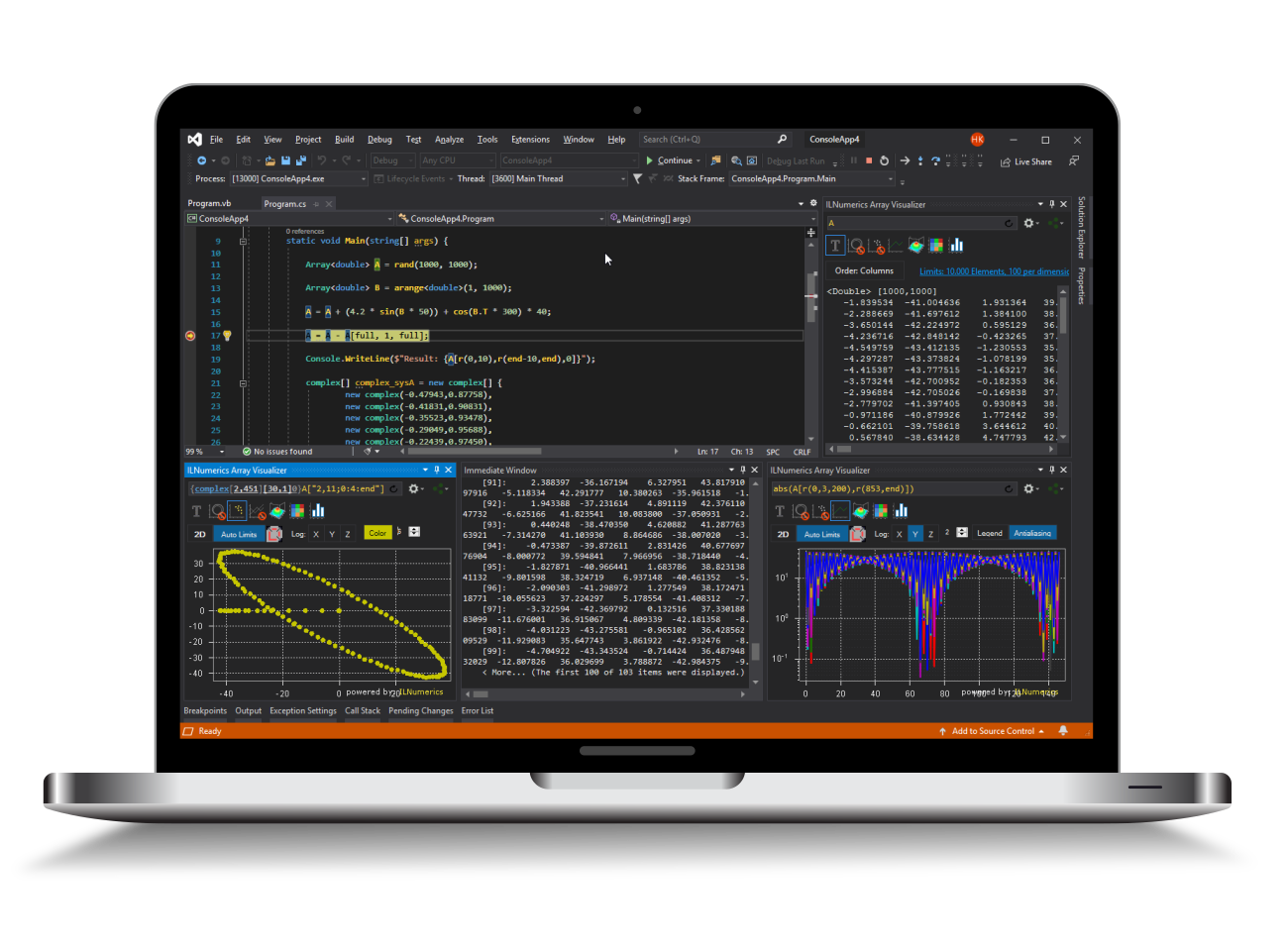Industrial Data Science
in C# and .NET:
Simple. Fast. Reliable.ILNumerics - Technical Computing

Modern High Performance Tools for Technical

Computing and Visualization in Industry and Science

tgt

# Logical Arrays

Logical arrays in ILNumerics are similar to regular arrays, having elements of type bool. Logicals are created as result of binary comparison operations (<,>,==,!=,>=,...) and unary test functions like any,isnan,isposinf, ...

Some examples of the creation of logical arrays:

The same options exist for handling logicals as for other arrays. Logicals can be used as local variable or returned from functions. However, logicals are mostly useful for the selection of elements and can directly be used on both sides of indexing expressions or as arguments of the Subarray() or SetRange() functions.

An expression A[L], where L is a logical array, is interpreted as A[find(L)]. I.e., nonzero elements of L are searched and given to the indexer (or Subarray()/ SetRange()) as column vector of sequential element indices. In order to return all even element values from A:

The scheme generalizes to the n-dimensional case. Logicals can be combined with all other common index specifiers for n-dimensional subarray range definitions. On the left side of assignments, logicals are convenient too:

## Number of True elements

Logical arrays track the number of their elements evaluating to 'true'. Being mostly an optimization feature the number can also be useful in algorithms: